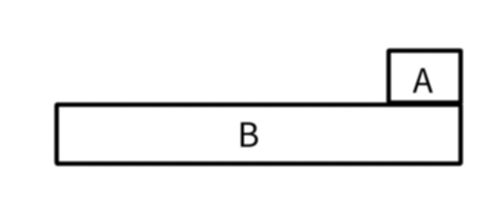# 一道动力学问题

07 / 31 / 2021 (最后编辑于 07 / 31 / 2021)

# 题目描述

​ (1) 试求刚开始运动时两实体的加速度。

​ (2) 若两个实体的模型互相重叠，而这种重叠并不是设计者原本的意图，我们称之为穿模现象。若实体 $A$ 的长度为 $l_A = 1m$ ，实体 $B$ 的长度为 $l_B = 22m$ ，高度为 $h_B = 1.25m$ ，现在由于游戏内容需要，小明同学需要在 $t = 2.5s$ 时取消两实体的碰撞箱，试分析两实体停止运动后会不会发生穿模现象（实体不会穿过水平地面）。（ $g = 10m/s^2$ )